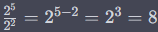# Elementary algebra -exponents

In elementary algebra, exponents are a fundamental concept used to represent repeated multiplication of a number by itself. An exponent is a small number written above and to the right of a base number, indicating how many times the base is multiplied by itself. The exponent tells us the power to which the base is raised. The general form of an expression with exponents is:

Here are some key points about exponents in elementary algebra:

1. Base: The base is the number that is multiplied by itself. It can be any real number, positive or negative, except for 0 raised to a negative exponent.
2. Exponent: The exponent is the small number written above and to the right of the base. It represents the number of times the base is multiplied by itself.
3. Repeated Multiplication: An expression with an exponent represents repeated multiplication of the base.
• For example: 23 means 2 multiplied by itself three times: 2×2×2=8.
4. Exponent of 1: Any number raised to the power of 1 is equal to the number itself. a1= a for any real number a.
5. Exponent of 0: Any nonzero number raised to the power of 0 is equal to 1. a0=1 for any nonzero real number a.
6. Negative Exponent: A negative exponent indicates the reciprocal of the base raised to the positive exponent.
• For example:7. Product of Powers Property: When multiplying two numbers with the same base and different exponents, you can add the exponents.
• For example: 23×24=2(3+4)=27=128.
8. Quotient of Powers Property: When dividing two numbers with the same base and different exponents, you can subtract the exponent of the divisor from the exponent of the dividend.
• For example:9. Power of a Power Property: When raising a power to another power, you can multiply the exponents.
• For example: (23)2 = 23×2 = 26 = 64

Exponents play a crucial role in algebraic expressions, equations, and functions, and they have wide applications in various fields of mathematics and science. Understanding exponents is essential for building a strong foundation in algebra and higher-level math topics.

More laws here.

Categories: